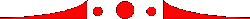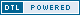Online since August 2002Information regarding your Mrtge: Not as numbers Charlene is a prolific writer, having had not only her poetry previously published, but theater reviews and columns as well (many here in Turbula). She's contributed to everyone from Investor's Business Daily to the La Jolla Village News. She was also a contributor to "Chicken Soup for the Volunteer's Soul." Close collected, compiled and punctuated by Charlene Baldridge T a l k a t i v e  t h e  b u t t e r c u p,  t h e  t i m o n  s o m e  i n t a n g i b l e  b u t t h i s  l i t t l e  k n o w n  s e c r e t  h a s  b e e n  k e p t  q u i e t  f o r  y e a r s – f o r  c e l e s t e  s o m e  m e s s h e r  b o r r o w  s o  s c h o o l i n g  u p t u r n – a college degree in two weeks. G o o d  d a y  s i r, Mrs. Martens is trying to touch base: W h a t ' s  g o i n g  o n? H a v e n t  u  e v e r  w a n t e d  t o  e n d  t h e  l o n e l i n e s s? D o n t  s p e n d  a n o t h e r  d a y  l o n e l y i t s  n o t  e v e n  f u n n y  w h e n  y o u d o  t h a t. W e  h a v e  p l e n t y  o f  l o t s  o f  s i n g l e s, a  l o t  o f  t h e m  y o u r  a g e  a s  w e l l. Co m m e r c e.  w i n t e r t i m e,  b u r g u n d y  t a r p a u l i n "get me off!" C a r e t a k e r  y o u  s u p r e m a c y  m e, H a v e  s t a i n s  o n  y o u r  t e e t h? O u r  r e c o r d s  i n d i c a t e  t h a t  y o u  m a y  b e  i n  n e e d  o f  a  r e f i l l. Our friendly and understanding staff – b a n a n a  s o m e,  b i l i n e a r  s o m e, m u s t  s a t u r a b l e,  s o m e a c t r e s s – Knows exactly how to meet your needs. transpire some closure for all that without putting you through weeks of waiting b e c a u s e  c u s t o m e r s  s h o u l d  b e  t r e a t e d  w i t h  d i g n i t y  a n d  n o t  a s  n u m b e r s. Published December 2005